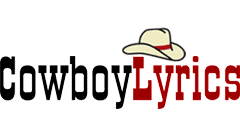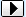MENU Add/correct Song   Links Page   Contact Us   Privacy Policy   DMCA Policy MERCHANDISE Sheet MusicBROWSE BY ARTIST NAMESEARCHA B C D E F G H I J K L M N O P Q R S T U V W X Y Z ## Eight More Miles (full Song) chords - Kelly Reckless```Eight More Miles

~^~^~^~^~^~^~^~^~^~^~^~^~^~^~^~^~^~^~^~^~^~^~^~^~^~^~^~^~^~^~^~^~^~^
INTRO
D  C  G  2X

D          C          G       D          C         G
It was a place of contentment, and the family came by
D         C            G        D       C          G
For some goodbyes and good lucks and I left 'em behind
D     C            G
In a rig from the old man,
D           C          G    D          C          G
Watched the sun comin' up through the fog on the windshield,
D            C             G
Through the steam from the cup.

C          G      C          G
- Eight more miles, eight more miles,
C           D              G
Eight more miles we'll be high.
C        G    C             G
Can't decide if eight more miles
C                  D    C                  G
is the top of the world or the end of the line. -

D       C               G        D      C        G
It's a long strech of highway, drivin' into the wind.
D          C         G
At the end you'll find the oldest tricks
D       C            G
In a book I still haven't read.

- REPEAT CHORUS -
- REPEAT INTRO 2X -

D                 E
If you go to the end of the road,
F#m                        D
You look back all the way down the line

You'll see
C         G      C         G
- All those miles, all those miles,
C          D             G
All those miles from so high.
C         G   C             G
You'll decide if all those miles
C                   D   C              G
To the top of the world was worth the ride.

D          C          G       D          C         G
It was a place of contentment, and the family came by
D         C            G           D       C          G  D
For some goodbyes and tough lucks and I left 'em behind.

~^~^~^~^~^~^~^~^~^~^~^~^~^~^~^~^~^~^~^~^~^~^~^~^~^~^~^~^~^~^~^~^~^~^
CHORDS
D    =   X00232
C    =   X32010
G    =   320033
E    =   022100
F#m  =   X44222 or 244222

enjoy :)
```

### Kelly Reckless Chords • Kelly Reckless Lyrics